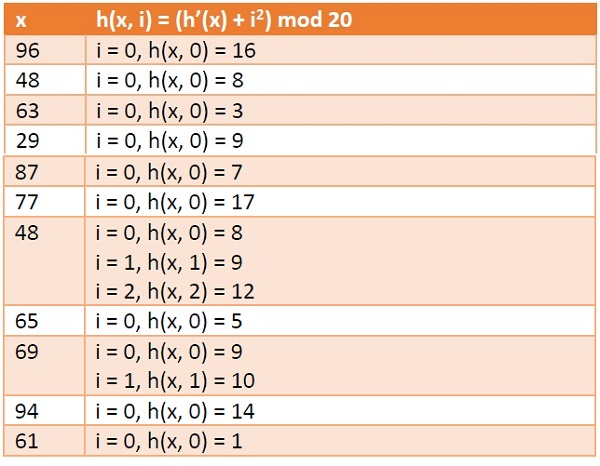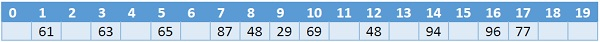# Quadratic Probing in Data Structure

Data StructureAnalysis of AlgorithmsAlgorithms

In this section we will see what is quadratic probing technique in open addressing scheme. There is an ordinary hash function h’(x) : U → {0, 1, . . ., m – 1}. In open addressing scheme, the actual hash function h(x) is taking the ordinary hash function h’(x) and attach some another part with it to make one quadratic equation.

h´ = (đť‘Ą) = đť‘Ą đť‘šđť‘śđť‘‘ đť‘š

â„Ž(đť‘Ą, đť‘–) = (â„Ž´(đť‘Ą) + đť‘–2)đť‘šđť‘śđť‘‘ đť‘š

We can put some other quadratic equations also using some constants

The value of i = 0, 1, . . ., m – 1. So we start from i = 0, and increase this until we get one free space. So initially when i = 0, then the h(x, i) is same as h´(x).

## Example

we have a list of size 20 (m = 20). We want to put some elements in linear probing fashion. The elements are {96, 48, 63, 29, 87, 77, 48, 65, 69, 94, 61}Hash Table